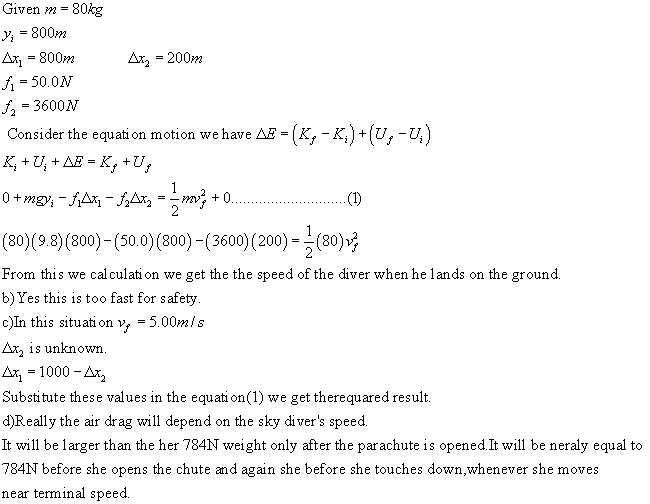# An 80.0-kg skydiver jumps out of a balloon at an altitude of1000 m and opens the parachute at an altitude of 200 m. (a) Assuming that the total retardmidtlinjeg 2020-10-21 Answered
An 80.0-kg skydiver jumps out of a balloon at an altitude of1000 m and opens the parachute at an altitude of 200 m. (a) Assuming that the total retarding force on the diver is constant at 50.0 N with the parachute closed and constant at 3600 N with the parachute open, what is the speed of the diver whenhe lands on the ground? (b) Do you think the skydiver will be injured? Explain. (c) At what height should the parachute be opened so that the final speed of the skydiver when he hits the ground is 5.00m/s? (d) How realistic is the assumption that the total retarding force is constant? Explain.
You can still ask an expert for help

• Questions are typically answered in as fast as 30 minutes

Solve your problem for the price of one coffee

• Math expert for every subject
• Pay only if we can solve itpierretteA.

###### Not exactly what you’re looking for?Jeffrey Jordon

a)Apply conservation of energy equation to the situation:

$\mathrm{△}K+\mathrm{△}U+{f}_{air}d=0$

Where the retarding force of air is the same as friction for our purpose.

$\left(\frac{1}{2}m{v}_{f}^{2}-\frac{1}{2}m{v}_{i}^{2}\right)+\left(mg{y}_{f}-mg{y}_{i}\right)+\left({f}_{1}{d}_{1}+{f}_{2}{d}_{2}\right)=0$

$\frac{1}{2}m{v}_{f}^{2}-mg{y}_{i}+{f}_{1}{d}_{1}+{f}_{2}{d}_{2}=0$

Solve for $\left({v}_{f}\right)$:

${v}_{f}=\sqrt{\frac{mg{y}_{i}-{f}_{1}{d}_{1}-{f}_{2}{d}_{2}}{m}}$

Substitute numerical values:

${v}_{f}=\sqrt{\frac{2\left[\left(80\right)\left(9.8\right)\left(1000\right)-\left(50\right)\left(800\right)-\left(3600\right)\left(200\right)\right]}{80}}$

=24.5 m/s

b) Yes, this speed is too fast when compared with the safety speed. So, he will definitely get injured.

c) Let (${d}_{2}$) be the distance above the ground when the parachute opens, therefore the initial distance of the skydiver is $\left(1000-{d}_{2}\right)$. Substitute those values in Eq.(*)

$\frac{1}{2}m{v}_{f}^{2}-mg{y}_{i}+{f}_{1}\left(1000-{d}_{2}\right)+{f}_{2}{d}_{2}=0$

$\frac{1}{2}m{v}_{f}^{2}-mg{y}_{i}+1000{f}_{1}-{f}_{1}{d}_{2}+{f}_{2}{d}_{2}=0$

$\frac{1}{2}m{v}_{f}^{2}-mg{y}_{i}+{d}_{2}\left({f}_{2}-{f}_{1}\right)+1000{f}_{1}=0$

Solve for (${d}_{2}$)

${d}_{2}=\frac{mg{y}_{i}-\frac{1}{2}m{v}_{f}^{2}-1000{f}_{1}}{{f}_{2}-{f}_{1}}$

Substitute numerical values:

${d}_{2}=\frac{\left(80\right)\left(9.8\right)\left(1000\right)-\frac{1}{2}\left(80\right)\left(5{\right)}^{2}-\left(1000\right)\left(50\right)}{3600-50}$

=206 m

d)

Not very realistic since air drag is proportional to the square of the speed. It will only be constant when the skydiver reaches terminal speed.